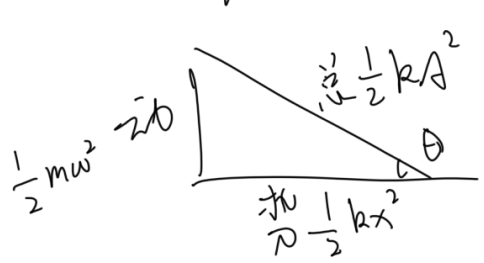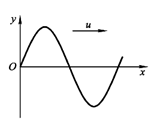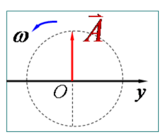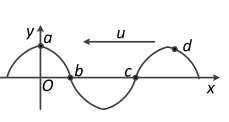【例子】 两个同振动方向、同频率、振幅均为 A 的简谐振动合成后，振幅仍为 A，则这两个简谐振动的相位差为（）

【分析与解答】

【例子】 求图中 $x= 0$ 处振动的初相位。【分析与解答】 $y = 0$，可知旋转矢量的相位是 $\pm \frac {\pi}{2}$ 将波形补充左边，并向 $u$ 的方向平移，可以发现 $x= 0$ 处的质点向 $y$ 负方向移动，也就是说，旋转矢量在下一时刻 $y$ 值要减少，所以只可能是 $\frac {\pi}{2}$【例子】 求图中 c 点的相位。【分析与解答】 可以发现 C 点 $y = 0$，所以相位是 $\pm \dfrac {\pi}{2}$，向左平移发现 $y$ 值变大，所以旋转矢量横坐标变大，应该是 $\dfrac {-\pi}{2}$，当然填个 $\dfrac {3}{2}\pi$ 也没毛病。